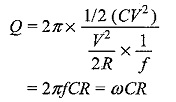## Quality Factor of Parallel RLC Circuit:

Consider the Quality Factor of Parallel RLC Circuit shown in Fig. 8.16.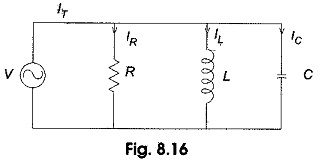In the circuit shown, the condition for resonance occurs when the susceptance part is zero.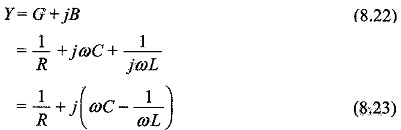The frequency at which resonance occurs is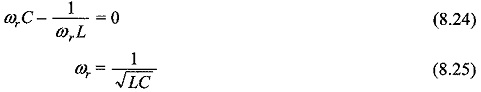The voltage and current variation with frequency is shown in Fig. 8.17. At resonant frequency, the current is minimum.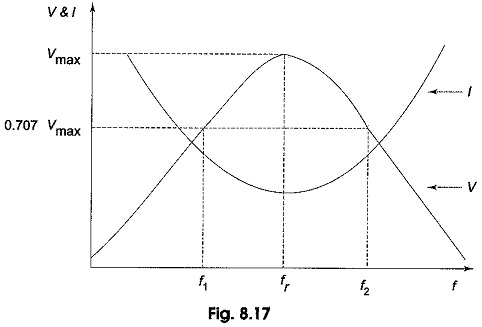The bandwidth,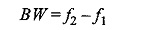For Parallel RLC Circuit, to obtain the lower half power frequency,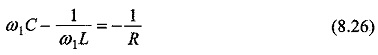From Eq. 8.26, we have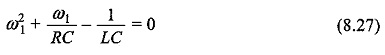If we simplify Eq. 8.27, we get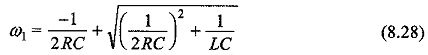Similarly, to obtain the upper half power frequency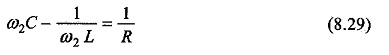From Eq. 8.29, we have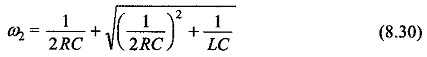Bandwidth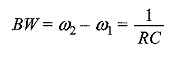The quality factor is defined as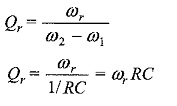In other words,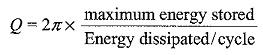In the case of an inductor,

The maximum energy stored = 1/2 LI2

Energy dissipated per cycle = (I/√2)2 x R x T

The quality factor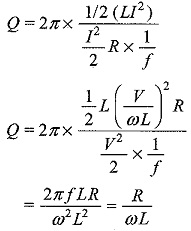For the capacitor, maximum energy stored = 1/2 (CV2)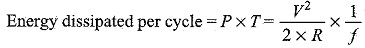The quality factor